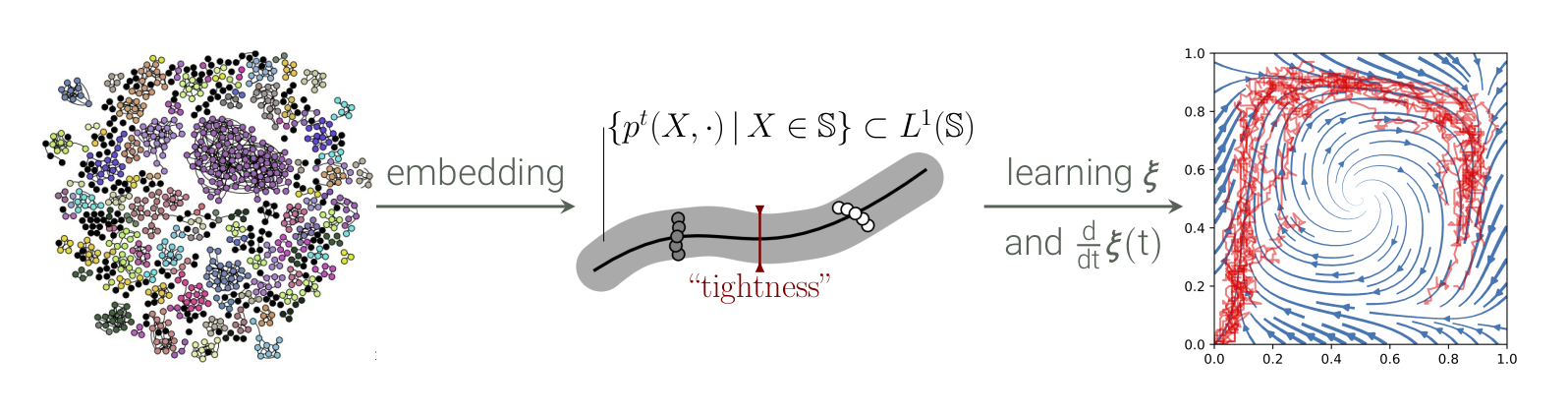Agent-based models (ABMs) are often high-dimensional and complex, making simulations costly and formal analysis hard. Low-dimensional model reduction is hence of great interest. The systems often show a “tightness”: the complex microdynamics of the O(N) many attributes of the individual agents can be approximated by the stochastic evolution of a small number (that is independent of N) of macroscopic collective variables describing the effective dynamics of the system. Moreover, if the number N of agents is large, one can observe a concentration of measure in the sense that the collective variables follow an almost deterministic and smooth evolution. In simple examples, suitable collective variables and approximate ODEs or SDEs governing the effective dynamics can be guessed or derived easily.

We formalize the efficacy of macroscopic modeling of ABMs by showing concentration of the process’ stochastic law transversal to low-dimensional coordinates of the full state. Further, we harness this property to numerically compute corresponding variables for systems where their analytical derivation is out of reach.